# Find the power of A for the matrix A = -1 0 0 0 - 1...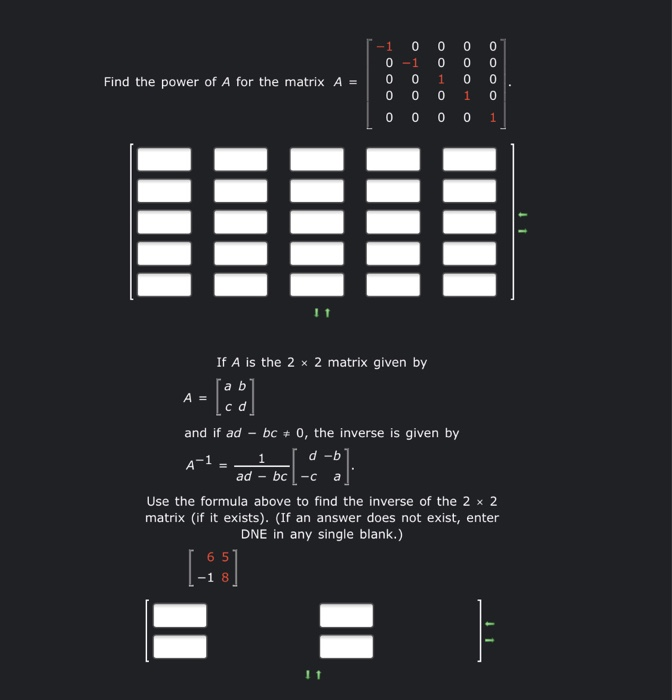Find the power of A for the matrix A = -1 0 0 0 - 1 0 0 0 0 OOOO OOOO 0 0 0 0 0 0 0 0 1 If A is the 2 x 2 matrix given by [aь A = cd and if ad - bc + 0, the inverse is given by d-b ad - bc Use the formula above to find the inverse of the 2 x 2 matrix (if it exists). (If an answer does not exist, enter DNE in any single blank.) 1 A-1 -C a 65 1-3: -1 8 ] 11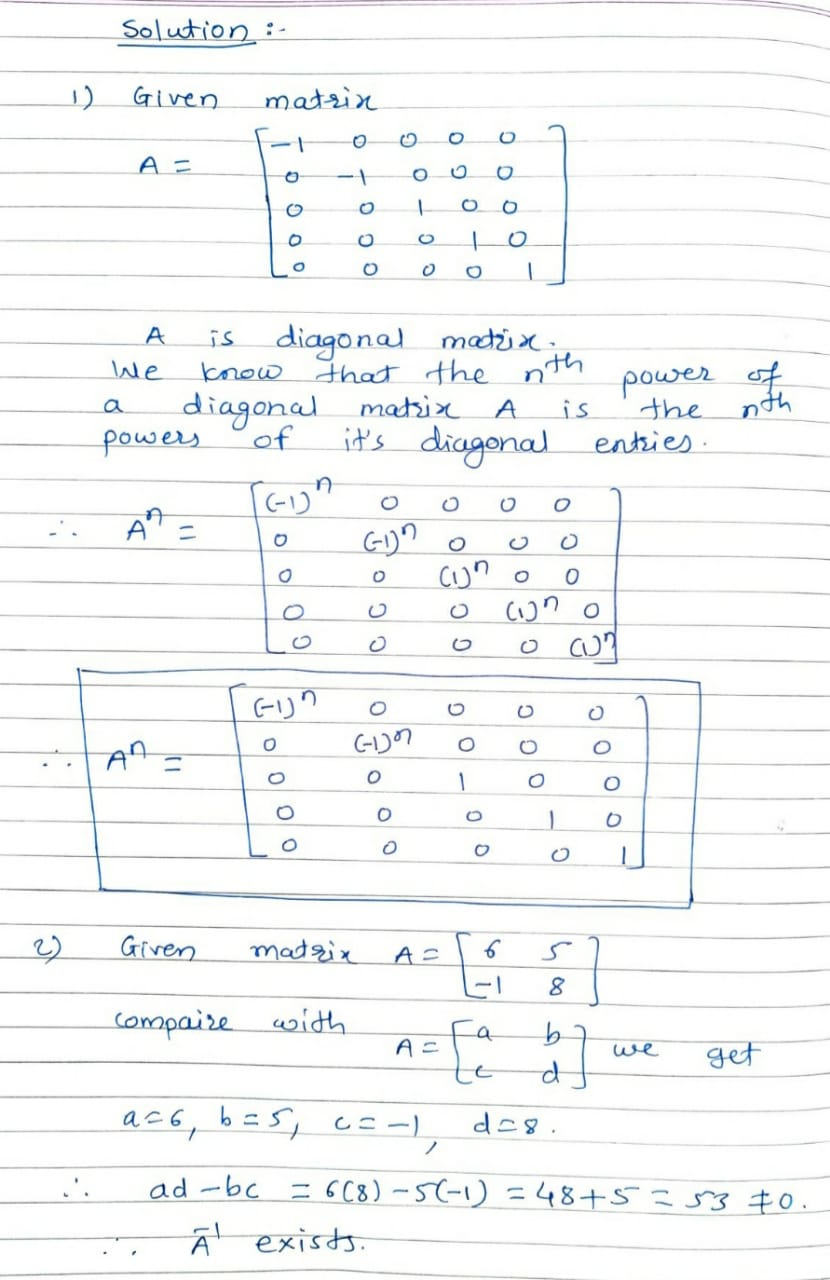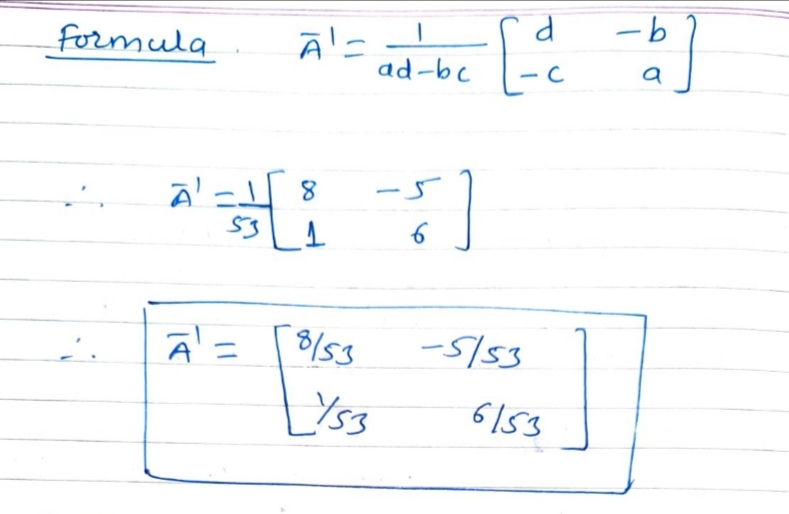#### Earn Coin

Coins can be redeemed for fabulous gifts.

Similar Homework Help Questions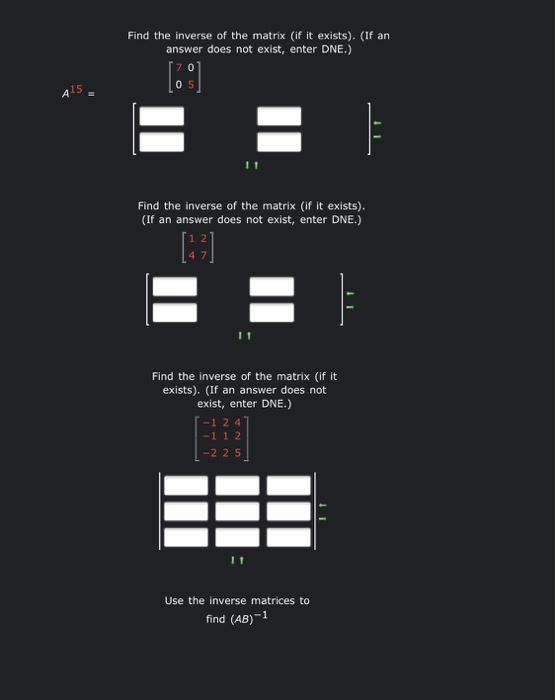3 part question about inverse of matrices. please help!! Find the inverse of the matrix (if it exists). (If an answer does not exist, enter DNE.)  05 415 E Find the inverse of the matrix (if it exists). (If an answer does not exist, enter DNE.) E = Find the inverse of the matrix (if it exists). (If an answer does not exist, enter DNE.) 1-1 2 4 -1 1 2 | -2 25 Use the inverse matrices to...

• ### Find the inverse of the matrix (if it exists). (If an answer does not exist, enter...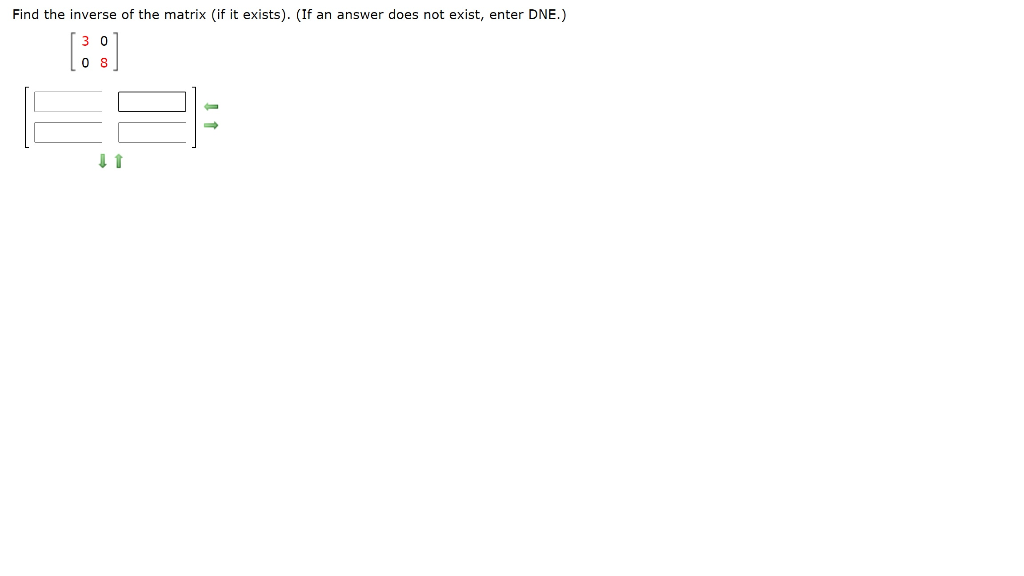Find the inverse of the matrix (if it exists). (If an answer does not exist, enter DNE.) 08 1 Find the inverse of the matrix (if it exists). (If an answer does not exist, enter DNE.)

• ### Find the inverse of the matrix (if it exists). (If an answer does not exist, enter...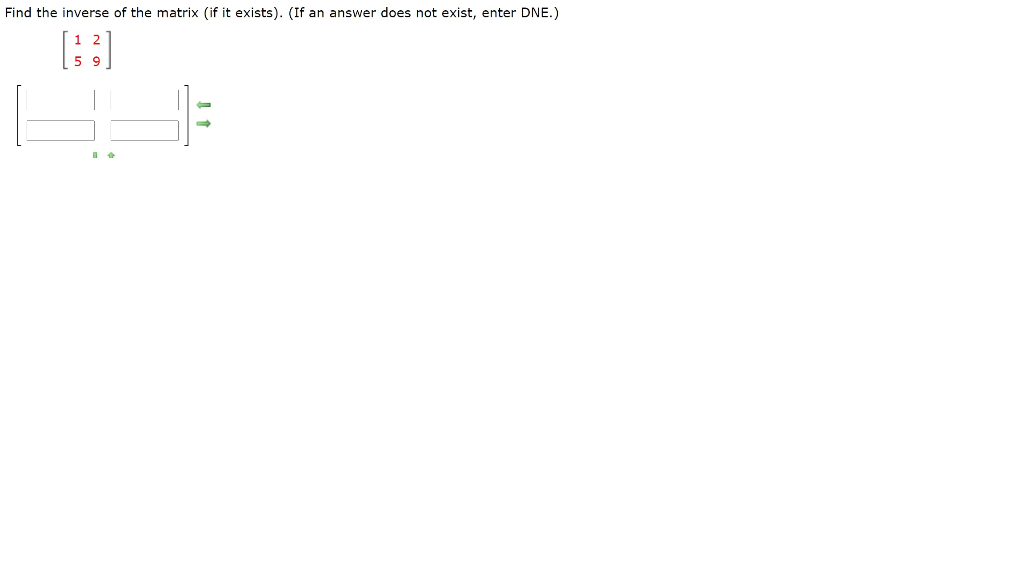Find the inverse of the matrix (if it exists). (If an answer does not exist, enter DNE.)

• ### Find the inverse of the matrix (if it exists). (If an answer does not exist, enter...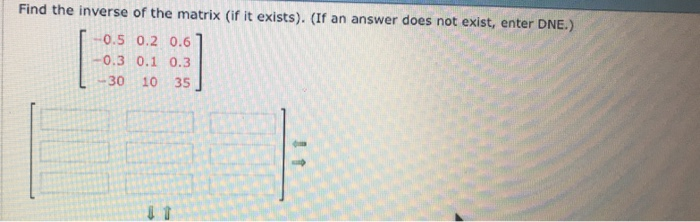Find the inverse of the matrix (if it exists). (If an answer does not exist, enter DNE.) 0.5 0.2 0.6 -0.3 0.1 0.3 L -30 10 35 J

• ### Linear Algebra Use the Quick Formula to find A1, if it exists. (If the inverse does...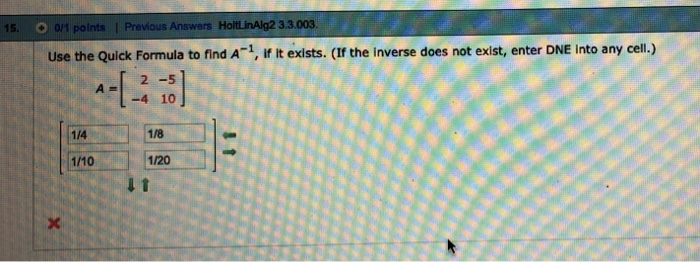Linear Algebra Use the Quick Formula to find A1, if it exists. (If the inverse does not exist, enter DNE Into any cell.) 2 -5 -4 10 1/4 1/8 1/10 1/20

• ### Find the inverse, if it exists, of the given matrix 1 0 0 OA. 0 1...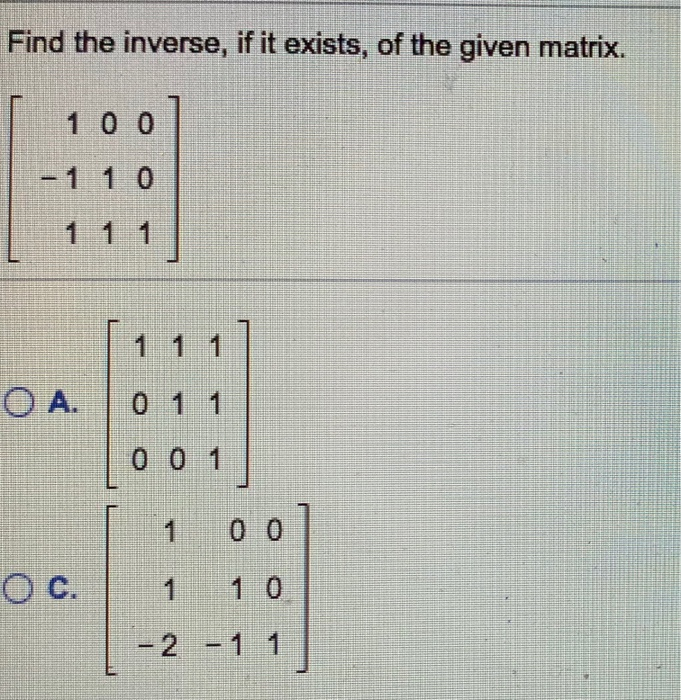Find the inverse, if it exists, of the given matrix 1 0 0 OA. 0 1 1 0 0 1 1 0 0 2-1 1 Find the inverse, if it exists, of the given matrix. 5 12 5 2 A. 12 5 5 -12 -2 5 -5 2 12 -5 -5-12 -25 OB. O c. O D. Determine whether the two matrices are inverses of each other by computing their product. 9 4-22 2 -45 O No O Yes

• ### Problem # 13 Please show all steps, formulas or any visuals necessary so that I may better understand how to approach other similar problems because I don't know how to get started, thank you. In...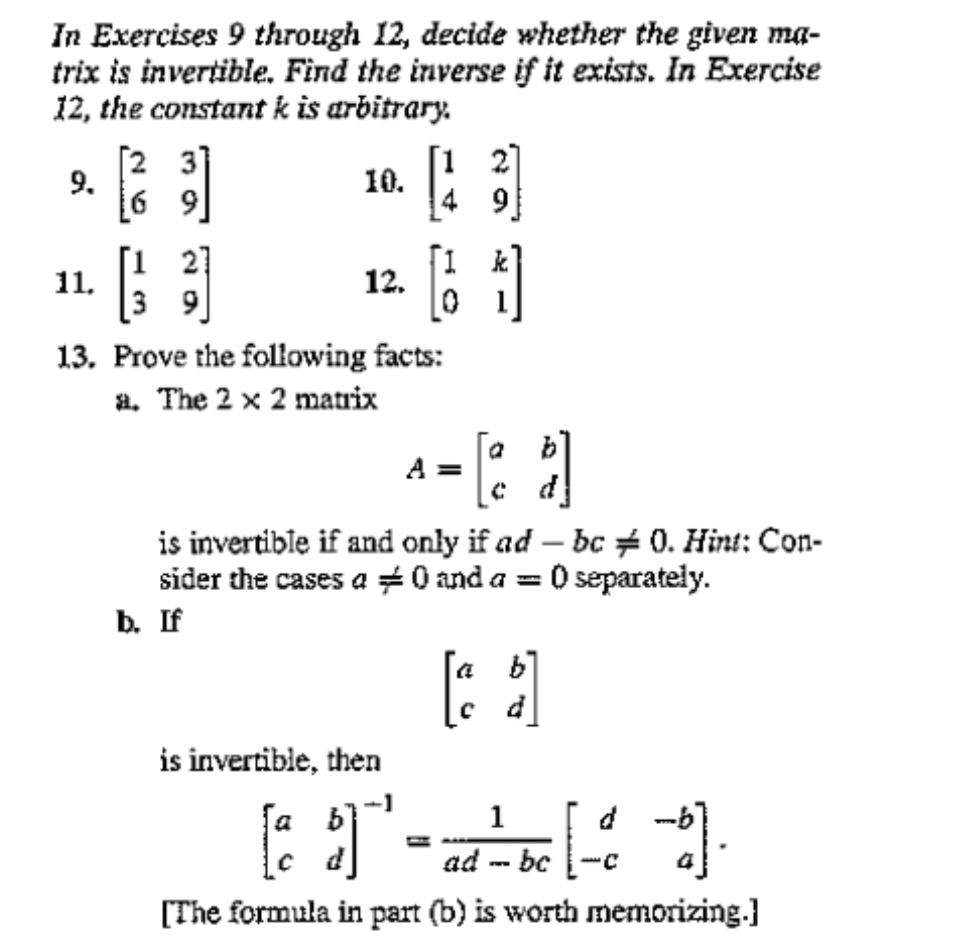Problem # 13 Please show all steps, formulas or any visuals necessary so that I may better understand how to approach other similar problems because I don't know how to get started, thank you. In Exercises 9 through I2, decide whether the given ma- trix is invertible. Find the inverse if it exists. In Exercise 12, the constant k is arbitrary. 9. 10. 12. 13. Prove the following facts: a. The 2 x 2 matrix is invertible if and only...

• ### Consider the following matrix 1 4 -1 1 A= 1 5 24 L2 8-22 Find a...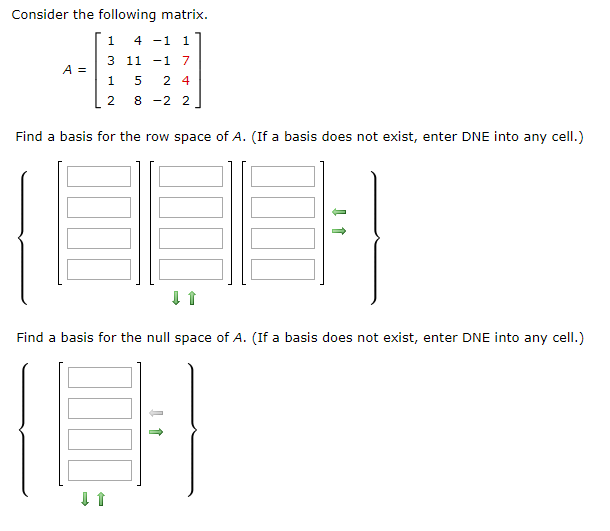Consider the following matrix 1 4 -1 1 A= 1 5 24 L2 8-22 Find a basis for the row space of A. (If a basis does not exist, enter DNE into any cell.) Find a basis for the null space of A. (If a basis does not exist, enter DNE into any cell.)

• ### 0 points) Matrix Operations - Inverse of a Matrix This problem is related to Problem 5.21...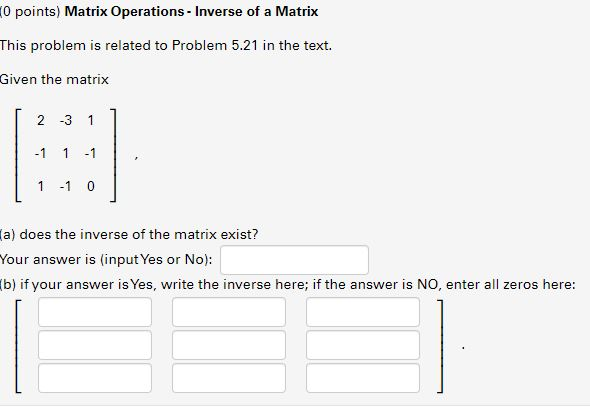0 points) Matrix Operations - Inverse of a Matrix This problem is related to Problem 5.21 in the text. Given the matrix I 2 -3 1 ] , -1 1 -1 1 -1 0 a) does the inverse of the matrix exist? Your answer is (input Yes or No): b) if your answer is Yes, write the inverse here; if the answer is NO, enter all zeros here:

• ### Need help with all 3 please. A = -3 0 6 1 7 5 and B...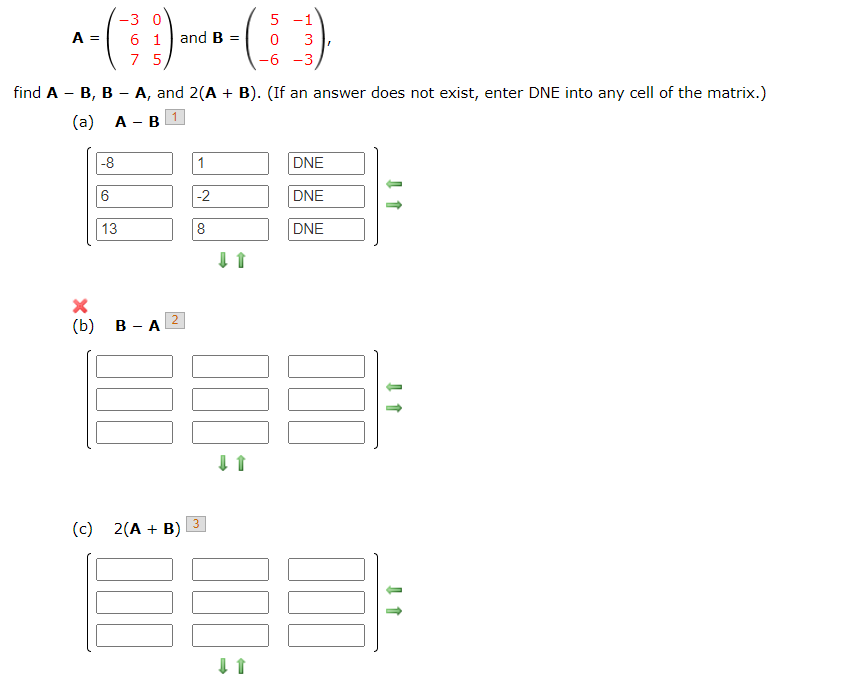Need help with all 3 please. A = -3 0 6 1 7 5 and B 5 -1 0 3 -6-3 find A - B, B - A, and 2(A + B). (If an answer does not exist, enter DNE into any cell of the matrix.) (a) A-B 1 -8 1 DNE 6 -2 DNE 13 8 DNE x (b) B-A 2 INT 3 (c) 2(A + B)